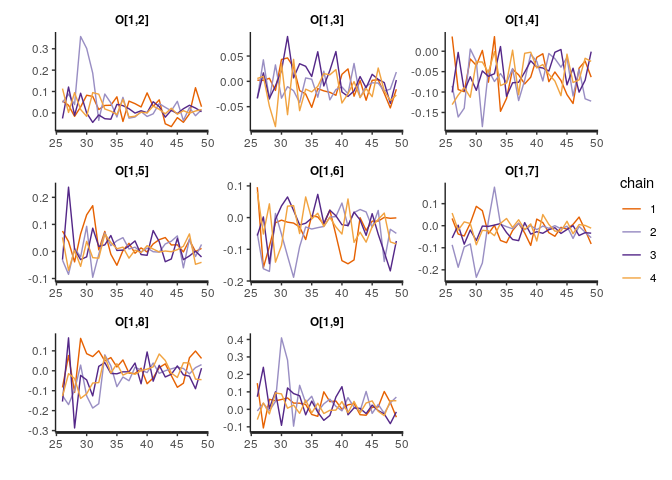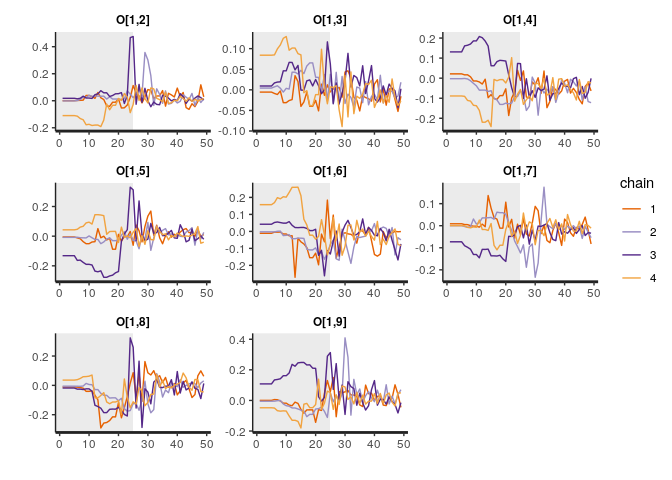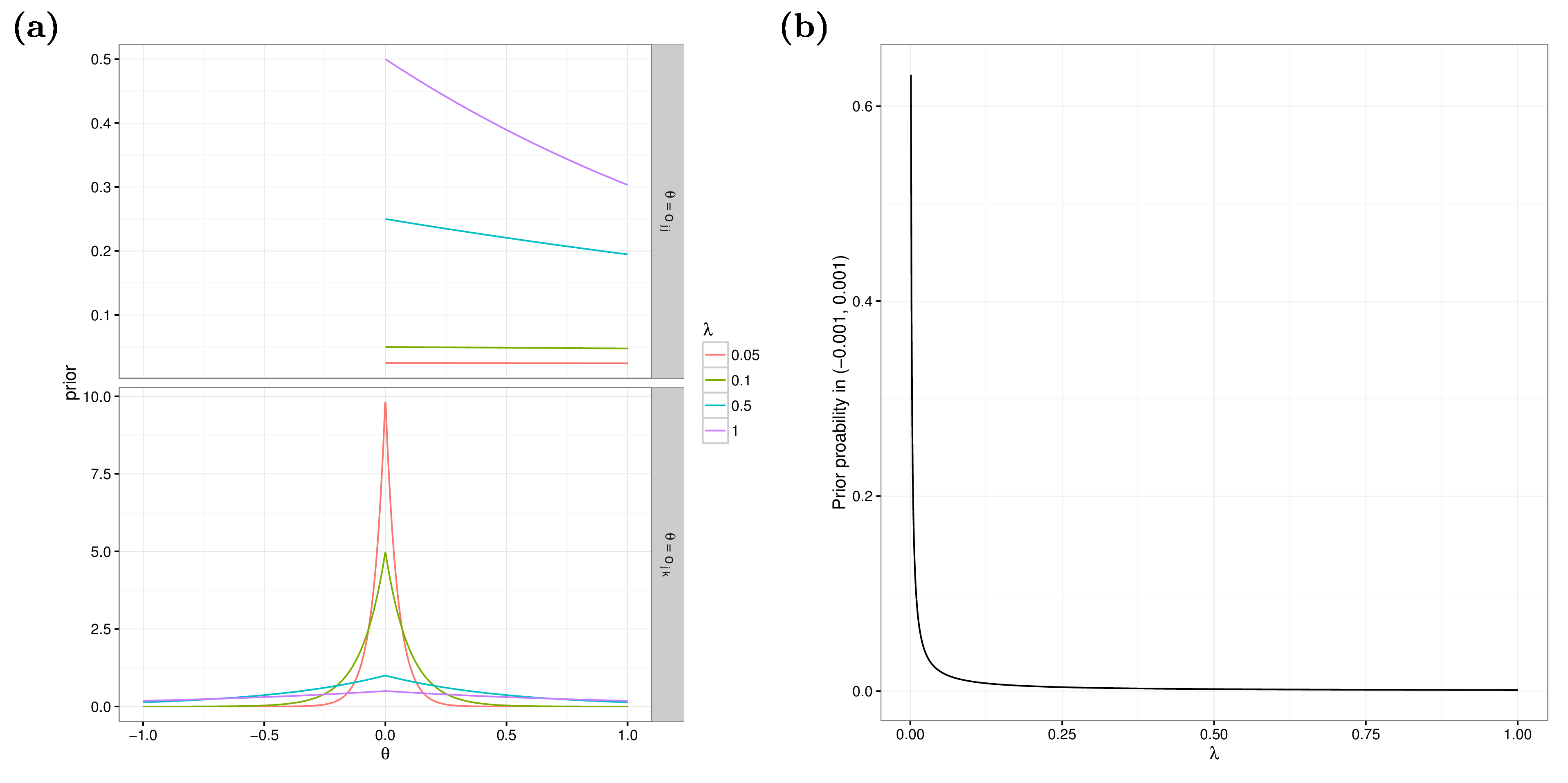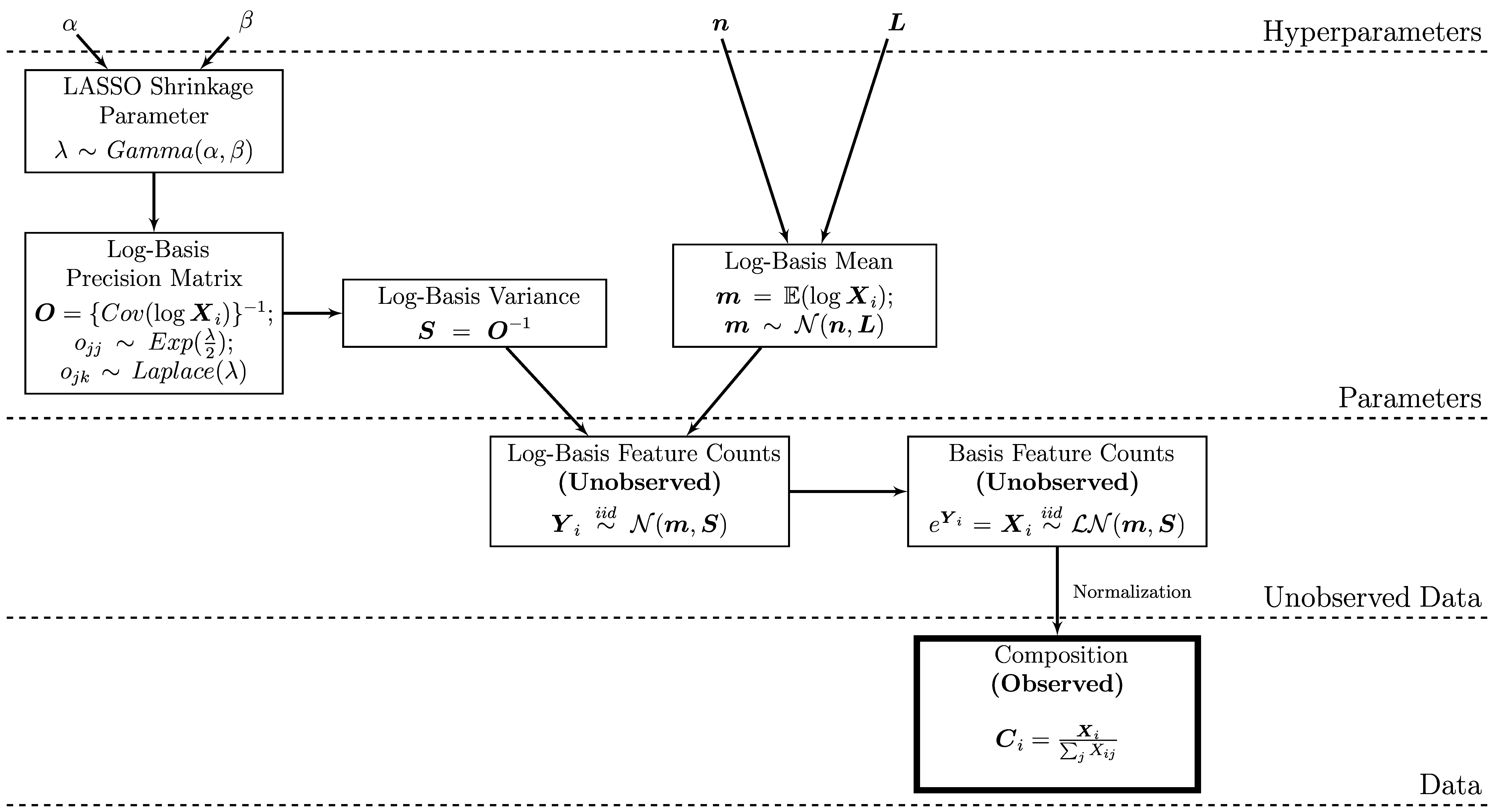# Introduction to BAnOCC (Bayesian Analaysis Of Compositional Covariance)

Emma Schwager 2019-05-02

## Introduction

Compositional data occur in many disciplines: geology, nutrition, economics, and ecology, to name a few. Data are compositional when each sample is sum-constrained. For example, mineral compositions describe a mineral in terms of the weight percentage coming from various elements; or taxonomic compositions break down a community by the fraction of community memebers that come from a particular species. In ecology in particular, the covariance between features is often of interest to determine which species possibly interact with each other. However, the sum constraint of compositional data makes naive measures inappropriate.

BAnOCC is a package for analyzing compositional covariance while accounting for the compositional structure. Briefly, the model assumes that the unobserved counts are log-normally distributed and then infers the correlation matrix of the log-basis (see the The Model section for a more detailed explanation). The inference is made using No U-Turn Sampling for Hamiltonian Monte Carlo (Hoffman and Gelman 2014) as implemented in the rstan R package (Stan Development Team 2015b).

## How To Install

There are three options for installing BAnOCC:

• Within R
• Using compressed file from bitbucket
• Directly from bitbucket

### From Within R

This is not yet available

### From Bitbucket (Compressed File)

This is not yet available

### From Bitbucket (Directly)

Clone the repository using git clone, which downloads the package as its own directory called banocc.

git clone https://<your-user-name>@bitbucket.org/biobakery/banocc.git

Then, install BAnOCC’s dependencies. If these are already installed on your machine, this step can be skipped.

Rscript -e "install.packages(c('rstan', 'mvtnorm', 'coda', 'stringr'))"

Lastly, install BAnOCC using R CMD INSTALL. Note that this will not automatically install the dependencies, so they must be installed first.

R CMD INSTALL banocc

## How To Run

### Loading

We first need to load the package:

library(banocc)
## Loading required package: rstan

## Loading required package: ggplot2

## Registered S3 methods overwritten by 'ggplot2':
##   method         from
##   [.quosures     rlang
##   c.quosures     rlang
##   print.quosures rlang

## Loading required package: StanHeaders

## rstan (Version 2.18.2, GitRev: 2e1f913d3ca3)

## For execution on a local, multicore CPU with excess RAM we recommend calling
## options(mc.cores = parallel::detectCores()).
## To avoid recompilation of unchanged Stan programs, we recommend calling
## rstan_options(auto_write = TRUE)


### Package Features

The BAnOCC package contains four things:

• banocc_model, which is the BAnOCC model in the rstan format

• run_banocc, a wrapper function for rstan::sampling that samples from the model and returns a list with various useful elements

• get_banocc_output, which takes as input a stanfit object outputted by run_banocc, and outputs various statistics from the chains.

• Several test datasets which are included both as counts and as the corresponding compositions:

Dataset Description Counts Composition
No correlations in the counts counts_null compositions_null
No correlations in the counts counts_hard_null compositions_hard_null
Positive corr. in the counts counts_pos_spike compositions_pos_spike
Negative corr. in the counts counts_neg_spike compositions_neg_spike

### Data and Prior Input

For a full and complete description of the possible parameters for run_banocc and get_banocc_output, their default values, and the output, see

?run_banocc
?get_banocc_output

#### Required Input

There are only two required inputs to run_banocc:

1. The dataset C. This is assumed to be (N \times P), with (N) samples and (P) features. The row sums are therefore required to be less than one for all samples.
2. The compiled stan model compiled_banocc_model. The compiled model is required so that run_banocc doesn’t need to waste time compiling the model every time it is called. To compile, use rstan::stan_model(model_code=banocc::banocc_model).

The simplest way to run the model is to load a test dataset, compile the model, sample from it (this gives a warning because the default number of iterations is low), and get the output:

Sys.setenv("PKG_CXXFLAGS"="-std=c++11")
data(compositions_null)
compiled_banocc_model <- rstan::stan_model(model_code = banocc::banocc_model)
b_fit     <- banocc::run_banocc(C = compositions_null, compiled_banocc_model=compiled_banocc_model)
## Warning: There were 8 divergent transitions after warmup. Increasing adapt_delta above 0.8 may help. See
## http://mc-stan.org/misc/warnings.html#divergent-transitions-after-warmup

## Warning: Examine the pairs() plot to diagnose sampling problems

## Warning: There were 2 chains where the estimated Bayesian Fraction of Missing Information was low. See
## http://mc-stan.org/misc/warnings.html#bfmi-low

## Warning: Examine the pairs() plot to diagnose sampling problems

## Warning in evaluate_convergence(b_stanfit = Fit, verbose = verbose,
## num_level = num_level + : Fit has not converged as evaluated by the Rhat
## statistic. You might try a larger number of warmup iterations, different
## priors, or different initial values. See vignette for more on evaluating
## convergence.

b_output <- banocc::get_banocc_output(banoccfit=b_fit)
## Warning in evaluate_convergence(b_stanfit = b_stanfit, verbose = verbose, :
## Fit has not converged as evaluated by the Rhat statistic. You might try a
## larger number of warmup iterations, different priors, or different initial
## values. See vignette for more on evaluating convergence.


#### Hyperparameters

The hyperparameter values can be specified as input to run_banocc. Their names correspond to the parameters in the plate diagram figure (see section The Model). For example,

Sys.setenv("PKG_CXXFLAGS"="-std=c++11")
p <- ncol(compositions_null)
b_fit_hp <- banocc::run_banocc(C = compositions_null,
compiled_banocc_model = compiled_banocc_model,
n = rep(0, p),
L = 10 * diag(p),
a = 0.5,
b = 0.01)
## Warning: There were 8 divergent transitions after warmup. Increasing adapt_delta above 0.8 may help. See
## http://mc-stan.org/misc/warnings.html#divergent-transitions-after-warmup

## Warning: Examine the pairs() plot to diagnose sampling problems

## Warning: There were 2 chains where the estimated Bayesian Fraction of Missing Information was low. See
## http://mc-stan.org/misc/warnings.html#bfmi-low

## Warning: Examine the pairs() plot to diagnose sampling problems

## Warning in evaluate_convergence(b_stanfit = Fit, verbose = verbose,
## num_level = num_level + : Fit has not converged as evaluated by the Rhat
## statistic. You might try a larger number of warmup iterations, different
## priors, or different initial values. See vignette for more on evaluating
## convergence.


### Sampling Control

There are several options to control the behavior of the HMC sampler within run_banocc. This is simply a call to rstan::sampling, and so many of the parameters are the same.

#### General Sampling Control

The number of chains, iterations, and warmup iterations as well as the rate of thinning for run_banocc can be specified using the same parameters as for rstan::sampling and rstan::stan. For example, the following code gives a total of three iterations from each of two chains. These parameters are used only for brevity and are NOT recommended in practice.

b_fit_sampling <- banocc::run_banocc(C = compositions_null,
compiled_banocc_model = compiled_banocc_model,
chains = 2,
iter = 11,
warmup = 5,
thin = 2)
## Warning: There were 4 divergent transitions after warmup. Increasing adapt_delta above 0.8 may help. See
## http://mc-stan.org/misc/warnings.html#divergent-transitions-after-warmup

## Warning: Examine the pairs() plot to diagnose sampling problems

## Warning: There were 6 divergent transitions after warmup. Increasing adapt_delta above 0.8 may help. See
## http://mc-stan.org/misc/warnings.html#divergent-transitions-after-warmup

## Warning: Examine the pairs() plot to diagnose sampling problems

## Warning in evaluate_convergence(b_stanfit = Fit, verbose = verbose,
## num_level = num_level + : Fit has not converged as evaluated by the Rhat
## statistic. You might try a larger number of warmup iterations, different
## priors, or different initial values. See vignette for more on evaluating
## convergence.


#### Number of Cores

The number of cores used for sampling on a multi-processor machine can also be specified, which allows chains to run in parallel and therefore decreases computation time. Since its purpose is running chains in parallel, computation time will decrease as cores are added up to when the number of cores and the number of chains are equal.

# This code is not run
b_fit_cores <- banocc::run_banocc(C = compositions_null,
compiled_banocc_model = compiled_banocc_model,
chains = 2,
cores = 2)

#### Initial Values

By default, the initial values for (m) and (\lambda) are sampled from the priors and the initial values for (O) are set to the identity matrix of dimension (P). Setting the initial values for (O) to the identity helps ensure a parsimonious model fit. The initial values can also be set to a particular value by using a list whose length is the number of chains and whose elements are lists of initial values for each parameter:

init <- list(list(m = rep(0, p),
O = diag(p),
lambda = 0.02),
list(m = runif(p),
O = 10 * diag(p),
lambda = runif(1, 0.1, 2)))
b_fit_init <- banocc::run_banocc(C = compositions_null,
compiled_banocc_model = compiled_banocc_model,
chains = 2,
init = init)
## Warning in evaluate_convergence(b_stanfit = Fit, verbose = verbose,
## num_level = num_level + : Fit has not converged as evaluated by the Rhat
## statistic. You might try a larger number of warmup iterations, different
## priors, or different initial values. See vignette for more on evaluating
## convergence.


More specific control of the sampler’s behavior comes from the control argument to rstan::sampling. Details about this argument can be found in the help for the rstan::stan function:

?stan

### Output Control

There are several parameters that control the type of output which is returned by get_banocc_output.

#### Credible Interval Width

The width of the returned credible intervals is controlled by conf_alpha. A (100% * (1-\alpha_\text{conf})) credible interval is returned:

# Get 90% credible intervals
b_out_90 <- banocc::get_banocc_output(banoccfit=b_fit,
conf_alpha = 0.1)
## Warning in evaluate_convergence(b_stanfit = b_stanfit, verbose = verbose, :
## Fit has not converged as evaluated by the Rhat statistic. You might try a
## larger number of warmup iterations, different priors, or different initial
## values. See vignette for more on evaluating convergence.

# Get 99% credible intervals
b_out_99 <- banocc::get_banocc_output(banoccfit=b_fit,
conf_alpha = 0.01)
## Warning in evaluate_convergence(b_stanfit = b_stanfit, verbose = verbose, :
## Fit has not converged as evaluated by the Rhat statistic. You might try a
## larger number of warmup iterations, different priors, or different initial
## values. See vignette for more on evaluating convergence.


#### Checking Convergence

Convergence is evaluated automatically, and in this case the credible intervals, estimates, and any additional output in section Additional Output is missing. This behavior can be turned off using the eval_convergence option. But be careful!

# Default is to evaluate convergence
b_out_ec <- banocc::get_banocc_output(banoccfit=b_fit)
## Warning in evaluate_convergence(b_stanfit = b_stanfit, verbose = verbose, :
## Fit has not converged as evaluated by the Rhat statistic. You might try a
## larger number of warmup iterations, different priors, or different initial
## values. See vignette for more on evaluating convergence.

# This can be turned off using eval_convergence
b_out_nec <- banocc::get_banocc_output(banoccfit=b_fit,
eval_convergence = FALSE)
# Iterations are too few, so estimates are missing
b_out_ec$Estimates.median ## f_n_1 f_n_2 f_n_3 f_n_4 f_n_5 f_n_6 f_n_7 f_n_8 f_n_9 ## f_n_1 NA NA NA NA NA NA NA NA NA ## f_n_2 NA NA NA NA NA NA NA NA NA ## f_n_3 NA NA NA NA NA NA NA NA NA ## f_n_4 NA NA NA NA NA NA NA NA NA ## f_n_5 NA NA NA NA NA NA NA NA NA ## f_n_6 NA NA NA NA NA NA NA NA NA ## f_n_7 NA NA NA NA NA NA NA NA NA ## f_n_8 NA NA NA NA NA NA NA NA NA ## f_n_9 NA NA NA NA NA NA NA NA NA  # Convergence was not evaluated, so estimates are not missing b_out_nec$Estimates.median
##              f_n_1        f_n_2         f_n_3        f_n_4        f_n_5
## f_n_1  1.000000000 -0.011910335  0.0137496306  0.055014052 -0.005497647
## f_n_2 -0.011910335  1.000000000 -0.0030261423  0.017076845 -0.003168672
## f_n_3  0.013749631 -0.003026142  1.0000000000 -0.018577815  0.044168591
## f_n_4  0.055014052  0.017076845 -0.0185778151  1.000000000 -0.003909243
## f_n_5 -0.005497647 -0.003168672  0.0441685913 -0.003909243  1.000000000
## f_n_6  0.014201814  0.003567740 -0.0005576013 -0.020029006 -0.007694461
## f_n_7  0.015139091  0.068932868  0.0180794763 -0.001538356  0.013329293
## f_n_8  0.002828996 -0.011808463 -0.0184771822  0.006720111  0.015514898
## f_n_9 -0.009973899  0.004383589  0.0086056004  0.050794660 -0.014471340
##               f_n_6        f_n_7        f_n_8        f_n_9
## f_n_1  0.0142018138  0.015139091  0.002828996 -0.009973899
## f_n_2  0.0035677402  0.068932868 -0.011808463  0.004383589
## f_n_3 -0.0005576013  0.018079476 -0.018477182  0.008605600
## f_n_4 -0.0200290061 -0.001538356  0.006720111  0.050794660
## f_n_5 -0.0076944611  0.013329293  0.015514898 -0.014471340
## f_n_6  1.0000000000  0.016483122  0.018034900 -0.006130580
## f_n_7  0.0164831224  1.000000000 -0.019505797 -0.002753455
## f_n_8  0.0180349002 -0.019505797  1.000000000  0.013898313
## f_n_9 -0.0061305795 -0.002753455  0.013898313  1.000000000


#### Additional Output

Two types of output can be requested for each correlation that are not included by default:

1. The smallest credible interval width that includes zero
2. The scaled neighborhood criterion, or SNC (Li and Lin 2010)
# Get the smallest credible interval width that includes zero
b_out_min_width <- banocc::get_banocc_output(banoccfit=b_fit,
get_min_width = TRUE)
## Warning in evaluate_convergence(b_stanfit = b_stanfit, verbose = verbose, :
## Fit has not converged as evaluated by the Rhat statistic. You might try a
## larger number of warmup iterations, different priors, or different initial
## values. See vignette for more on evaluating convergence.

# Get the scaled neighborhood criterion
b_out_snc <- banocc::get_banocc_output(banoccfit=b_fit,
calc_snc = TRUE)
## Warning in evaluate_convergence(b_stanfit = b_stanfit, verbose = verbose, :
## Fit has not converged as evaluated by the Rhat statistic. You might try a
## larger number of warmup iterations, different priors, or different initial
## values. See vignette for more on evaluating convergence.


Detailed statements about the function’s execution can also be printed using the verbose argument. The relative indentation of the verbose output indicates the nesting level of the function. The starting indentation can be set with num_level.

## Assessing Convergence

There are many ways of assessing convergence, but the two most easily implemented using BAnOCC are:

1. Traceplots of parameters, which show visually what values of a parameter have been sampled across all iterations. At convergence, the sampler should be moving rapidly across the space, and the chains should overlap well. In other words, it should look like grass.

2. The Rhat statistic (Gelman and Rubin 1992), which measures agreement between all the chains. It should be close to one at convergence.

### Traceplots

Traceplots can be directly accessed using the traceplot function in the rstan package, which creates a ggplot2 object that can be further maniuplated to ‘prettify’ the plot. The traceplots so generated are for the samples drawn after the warmup period. For example, we could plot the traceplots for the inverse covariances of feature 1 with all other features. There is overlap between some of the chains, but not all and so we conclude that we need more samples from the posterior to be confident of convergence.

# The inverse covariances of feature 1 with all other features
rstan::traceplot(b_fit$Fit, pars=paste0("O[1,", 2:9, "]"))We could also see the warmup period samples by using inc_warmup=TRUE. This shows that some of the chains have moved from very different starting points to a similar distribution, which is a good sign of convergence. # The inverse covariances of feature 1 with all other features, including warmup rstan::traceplot(b_fit$Fit, pars=paste0("O[1,", 2:9, "]"),
inc_warmup=TRUE)### Rhat Statistics

The Rhat values can also be directly accessed using the summary function in the rstan package. It measures the degree of agreement between all the chains. At convergence, the Rhat statistics should be approximately one for all parameters. For example, the Rhat values for the correlation between feature 1 and all other features (the same as those plotted above), agree with the traceplots that convergence has not yet been reached.

# This returns a named vector with the Rhat values for all parameters
rhat_all <- rstan::summary(b_fit$Fit)$summary[, "Rhat"]

# To see the Rhat values for the inverse covariances of feature 1
rhat_all[paste0("O[1,", 2:9, "]")]
##   O[1,2]   O[1,3]   O[1,4]   O[1,5]   O[1,6]   O[1,7]   O[1,8]   O[1,9]
## 1.085069 1.020772 1.001396 1.007636 1.040813 1.011966 1.095660 1.007823


## Choosing Priors

The hyperparameters for the model (see section The Model) need to be chosen appropriately.

### Log-Basis Precision Matrix

The prior on the precision matrix (O) is a GLASSO prior from (Wang 2012) with parameter (\lambda) [see also section The Model]. As (\lambda) decreases, the degree of shrinkage correspondingly increases.### Log-Basis Mean

We recommend using an uninformative prior for the log-basis mean: centered at zero and with large variance.

### GLASSO Shrinkage Parameter

We recommend using a prior with large probability mass close to zero; because (\lambda) has a gamma prior, this means that the shape parameter (a) should be less than one. The rate parameter (b) determines the variability; in cases with either small (order of 10) or very large ((p > n)) numbers of features (b) should be large so that the variance of the gamma distribution, (a / b^2), is small. Otherwise, a small value of (b) will make the prior more uninformative.

## The Model

A pictoral representation of the model is shown below. Briefly, the basis (or unobserved, unrestricted counts) for each sample is assumed to be a lognormal distribution with parameters (m) and (S). The prior on (m) is a normal distribution parametrized by mean (n) and variance-covariance matrix (L). Since we are using a graphical LASSO prior, we parametrize the model with precision matrix (O). The prior on (O) is a graphical LASSO prior (Wang 2012) with shrinkage parameter (\lambda). To circumvent the necessity of choosing (\lambda), a gamma hyperprior is placed on (\lambda), with parameters (a) and (b).If we print the model, we can actually see the code. It is written in the format required by the rstan package, since banocc uses this package to sample from the model. See (Stan Development Team 2015a) for more detailed information on this format.

# This code is not run
cat(banocc::banocc_model)

Gelman, Andrew, and Donald B. Rubin. 1992. “Inference from Iterative Simulation Using Multiple Sequences.” Statistical Science 7 (4). Institute of Mathematical Statistics:457–72. http://dx.doi.org/10.2307/2246093.

Hoffman, Matthew D., and Andrew Gelman. 2014. “The No-U-Turn Sampler: Adaptively Setting Path Lengths in Hamiltonian Monte Carlo.” J. Mach. Learn. Res. 15 (1). JMLR.org:1593–1623. http://dl.acm.org/citation.cfm?id=2627435.2638586.

Li, Qing, and Nan Lin. 2010. “The Bayesian Elastic Net.” Bayesian Anal. 5 (1). International Society for Bayesian Analysis:151–70. http://dx.doi.org/10.1214/10-BA506.

Stan Development Team. 2015a. Stan Modeling Language Users Guide and Reference Manual, Version 2.10.0. http://mc-stan.org/.

———. 2015b. “Stan: A C++ Library for Probability and Sampling, Version 2.10.0.” http://mc-stan.org/.

Wang, Hao. 2012. “Bayesian Graphical Lasso Models and Efficient Posterior Computation.” Bayesian Anal. 7 (4). International Society for Bayesian Analysis:867–86. https://doi.org/10.1214/12-BA729.